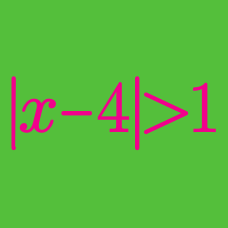Algebra

# Absolute Value Inequalities - 3 or More Linear Terms

What is the smallest positive integer $$n$$, such that there exists $$n$$ real numbers $$\{ x_i \} _{i=1}^n$$ which satisfy $$| x_i | < 1$$ and

$| x_1| + |x_2| + |x_3| + \ldots + |x_n| = 165 + |x_1 + x_2 + \ldots + x_n| ?$

As $$x$$ ranges over all real numbers, what is the smallest value of

$f(x) = |x- 165 | + |x-247 | + |x- 316 | ?$

Solve for $$x:$$ $\lvert x+16 \rvert - 2\lvert x - 16 \rvert + \lvert x \rvert > 4 .$

Six children are standing along the $$x$$-axis at points $$(0,0)$$, $$(30,0)$$, $$(87,0)$$, $$(142,0)$$, $$(237,0)$$, $$(504,0)$$. The children decide to meet at some point along the $$x$$-axis. What is the minimum total distance the children must walk in order to meet?

As $$x$$ ranges over all real values, what is the minimum value of $$f(x)=|x-333|+|x-631| + |x-856|$$?

Details and assumptions

The notation $$| \cdot |$$ denotes the absolute value. The function is given by $|x | = \begin{cases} x & x \geq 0 \\ -x & x < 0 \\ \end{cases}$ For example, $$|3| = 3, |-2| = 2$$.

×

Problem Loading...

Note Loading...

Set Loading...